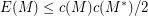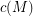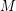# Equality in a matroidal circumference bound

 Importance: Medium ✭✭
 Author(s): Oxley, James Royle, Gordon
 Subject: Combinatorics » Matroid Theory
 Keywords: circumference
 Posted by: Gordon Royle on: November 2nd, 2007
Question   Is the binary affine cubethe only 3-connected matroid for which equality holds in the boundwhereis the circumference (i.e. largest circuit size) of?

Ifis a 2-connected matroid with at least two elements then it was proved in [LO] thatwhereis the size of the largest circuit in.

Equality can hold in this bound -- in particular the binary affine cubeis an 8-element self-dual matroid with circumference 4. There are various graphic matroids for which equality holds, and these have been classified in [W] where it is shown that they are all series-parallel networks and hence not 3-connected.

This question is therefore asking whetheris the sole-connected example where equality holds; this is known to be true for all matroids on up to 9 elements.

(A variant of this question would be to ask ifis the only non-graphic example other than trivial modifications like replacing every element with an equally sized parallel class.)

## Bibliography

[LO] Lemos, Manoel; Oxley, James A sharp bound on the size of a connected matroid. Trans. Amer. Math. Soc. 353 (2001), no. 10, 4039--4056 MathSciNet

[W] Wu, Pou-Lin Extremal graphs with prescribed circumference and cocircumference. Discrete Math. 223 (2000), no. 1-3, 299--308 MathSciNet

* indicates original appearance(s) of problem.